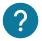# What is a float's variable ? How decimal numbers works on Arduino? How to make operation with it?

## Introduction

The float variables are used to store decimals numbers in your program.It correspond of all the positive and negative numbers with semicolon.

Here is how we declare a decimal number:

``float decimal_number = 3.14159;``

## Arithmetic operation

On these decimals numbers, you can do arithmetical operations such addition, substraction and much more. Here is some examples:

``````void setup() {
Serial.begin(9600); // We initialize the serial monitor
float variable_a=13.34; // The first variable
float variable_b=2.56; // The second variable
Serial.println(variable_a+variable_b); // We display the result of the addition
}

void loop() {
}``````
• Substraction
``````void setup() {
Serial.begin(9600); // We initialize the serial monitor
float variable_a=-5.34; // The first variable
float variable_b=8.46; // The second variable
Serial.println(variable_a-variable_b); // We display the result of the substraction
}

void loop() {
}``````
Here is a table gathering all the operations possible on decimal numbers:

TABLEAU

• The maximum between two decimal numbers

The maximum between two decimal numbers can be useful to return the most important value of the two. We will see an example below:

``````void setup() {
Serial.begin(9600); // We initialize the serial monitor
float variable_a=2.3; // We initialize the first variable
float variable_b=7.2; // We initialize the second variable
float variable_max=max(variable_a,variable_b); //  The variable_max return the maximum of the two variables
Serial.print("The maximum is:"); // We display the maximum between two numbers
Serial.println(variable_max);
}

void loop() {
}``````
You can also compare two decimal numbers in a if, for or while loop with comparison operators or even square root of a decimal number. You can find theses operators on our course mathematical functions.### How to convert an integer's variable or string's variable to a float's variable?

It is possible that you need to transform an variable int, string to a float. To do that you just have to add (float) before the variable to transform it to a decimal number.

Example with an integer variable:

``````void setup() {
Serial.begin(9600); // We initialize the serial monitor
int variable =23; //your float's variable
float variable_integer = (float)variable; // To convert the integer's variable
Serial.println(variable_integer); // We display the result
}

void loop() {
}``````

Example with a string variable:

``````void setup() {
Serial.begin(9600); // We initialize the serial monitor
string variable ="23.6"; //your float's variable
float variable_float = (float)variable; // To convert the string's variable
Serial.println(variable_float); // We display the result
}

void loop() {
}``````